# E-PolyLearning

 1. Choose the box that is similar to the box formed from the given sheet of paper (X).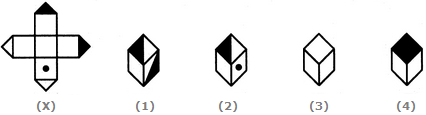a. 1 and 2 only b. 2 and 4 only c. 2 and 3 only d. 1 and 4 only

 2. Choose the box that is similar to the box formed from the given sheet of paper (X).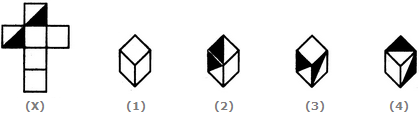a. 1 and 4 only b. 3 and 4 only c. 1 and 2 only d. 2 and 3 only
 3. How many dots lie opposite to the face having three dots, when the given figure is folded to form a cube?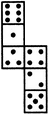a. 2 b. 4 c. 5 d. 6
 4. Choose the box that is similar to the box formed from the given sheet of paper (X).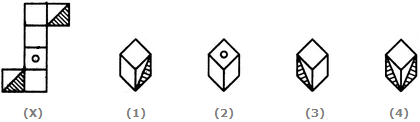a. 1 and 3 only b. 1 and 4 only c. 2 and 4 only d. 3 and 4 only
 5. Choose the box that is similar to the box formed from the given sheet of paper (X).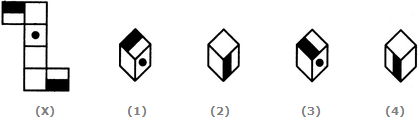a. 1 and 2 only b. 2 and 3 only c. 2 and 4 only d. 1, 2, 3 and 4